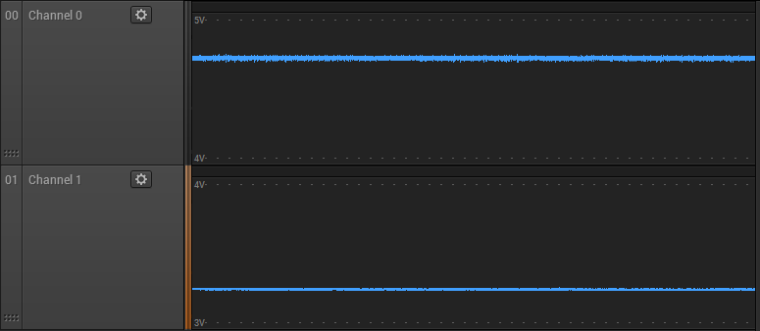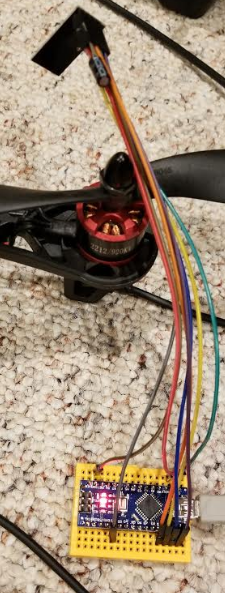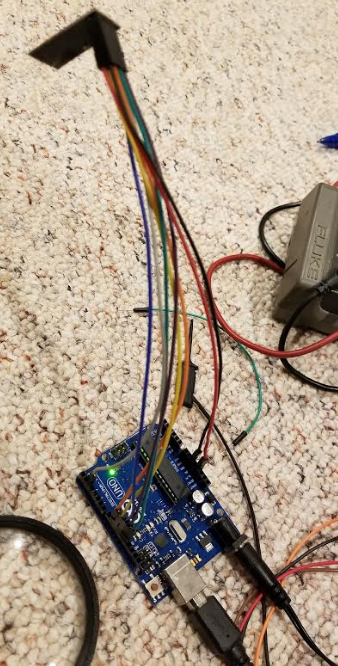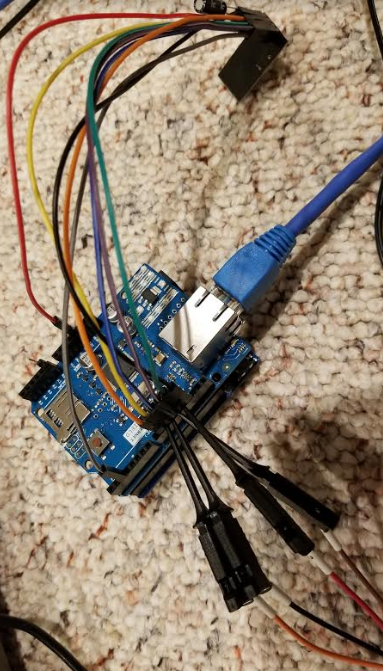# NACK Errors w/ Arduino Uno Ethernet Gateway v2.2.0 rc2

• Greetings!

I am putting a gateway and sensor together for the first time (about a week in). Long time user of Vera Plus and I have written my own code for it. Vera Plus is my controller. So far the MySensors experience has gone well with only a few things I had to figure out. But I do have an issue I haven't been able to solve.

In short, for a sensor node, which is an Arduino Nano w/ NRF24 radio (NRF24L01+), when it communicates to a gateway that is an Arduino Uno w/ a W5100 Ethernet shield and NRF24 radio, I see some NACK errors, especially at startup. The errors are enough to disrupt the proper operation of the node.

But, I also have a gateway which is a RPi (a B+ 1.2 I think) also with a NRF24 radio. The RPi runs the latest (as of this writing) 2017-11-29-raspbian-stretch. With the RPi acting as the gateway to the controller, the sensor node works perfectly with no NACK errors.

Both controllers and the sensor node are running the latest MySensors development version 2.2.0 rc2. The node itself simply provides a basic simulated door sensor. Basically it's a non-blocking loop that opens and closes the door every 10 seconds. The door sensor ID is 123 in the logs.

This is the log from the sensor node running with the Uno/Ethernet gateway. The NACK errors are evident.

`````` __  __       ____
|  \/  |_   _/ ___|  ___ _ __  ___  ___  _ __ ___
| |\/| | | | \___ \ / _ \ `_ \/ __|/ _ \| `__/ __|
| |  | | |_| |___| |  __/ | | \__ \  _  | |  \__ \
|_|  |_|\__, |____/ \___|_| |_|___/\___/|_|  |___/
|___/                      2.2.0-rc.2

17 MCO:BGN:INIT NODE,CP=RNNNA---,VER=2.2.0-rc.2
26 MCO:BGN:BFR
27 TSM:INIT
29 TSF:WUR:MS=0
35 TSM:INIT:TSP OK
37 TSF:SID:OK,ID=1
39 TSM:FPAR
75 TSF:MSG:SEND,1-1-255-255,s=255,c=3,t=7,pt=0,l=0,sg=0,ft=0,st=OK:
1086 TSF:MSG:FPAR OK,ID=0,D=1
2082 TSM:FPAR:OK
2083 TSM:ID
2084 TSM:ID:OK
2086 TSM:UPL
2092 TSF:MSG:SEND,1-1-0-0,s=255,c=3,t=24,pt=1,l=1,sg=0,ft=0,st=OK:1
2106 TSF:MSG:PONG RECV,HP=1
2108 TSM:UPL:OK
2125 TSF:MSG:SEND,1-1-0-0,s=255,c=3,t=15,pt=6,l=2,sg=0,ft=0,st=OK:0100
2142 TSF:MSG:SEND,1-1-0-0,s=255,c=0,t=17,pt=0,l=10,sg=0,ft=0,st=OK:2.2.0-rc.2
2182 TSF:MSG:SEND,1-1-0-0,s=255,c=3,t=6,pt=1,l=1,sg=0,ft=0,st=OK:0
2275 !TSF:MSG:SEND,1-1-0-0,s=255,c=3,t=11,pt=0,l=17,sg=0,ft=0,st=NACK:ArduinoDoorSensor
2323 !TSF:MSG:SEND,1-1-0-0,s=255,c=3,t=12,pt=0,l=11,sg=0,ft=1,st=NACK:Version 1.x
2332 TSF:MSG:SEND,1-1-0-0,s=123,c=0,t=0,pt=0,l=0,sg=0,ft=2,st=OK:
2338 MCO:REG:REQ
2376 !TSF:MSG:SEND,1-1-0-0,s=255,c=3,t=26,pt=1,l=1,sg=0,ft=0,st=NACK:2
4397 TSF:MSG:SEND,1-1-0-0,s=255,c=3,t=26,pt=1,l=1,sg=0,ft=1,st=OK:2
4408 MCO:PIM:NODE REG=1
4410 MCO:BGN:STP
4412 MCO:BGN:INIT OK,TSP=1
14418 TSF:MSG:SEND,1-1-0-0,s=123,c=1,t=16,pt=2,l=2,sg=0,ft=0,st=OK:1
24463 !TSF:MSG:SEND,1-1-0-0,s=123,c=1,t=16,pt=2,l=2,sg=0,ft=0,st=NACK:0
34471 TSF:MSG:SEND,1-1-0-0,s=123,c=1,t=16,pt=2,l=2,sg=0,ft=1,st=OK:1
``````

The log above continues with the events happening every 10 seconds. Roughly every 5 to 20 messages will result in a NACK, each an apparent a lost message or event.

This is the sensor node log running with the RPi/Ethernet gateway. There are no errors.

``````  __  __       ____
|  \/  |_   _/ ___|  ___ _ __  ___  ___  _ __ ___
| |\/| | | | \___ \ / _ \ `_ \/ __|/ _ \| `__/ __|
| |  | | |_| |___| |  __/ | | \__ \  _  | |  \__ \
|_|  |_|\__, |____/ \___|_| |_|___/\___/|_|  |___/
|___/                      2.2.0-rc.2

17 MCO:BGN:INIT NODE,CP=RNNNA---,VER=2.2.0-rc.2
26 MCO:BGN:BFR
27 TSM:INIT
29 TSF:WUR:MS=0
35 TSM:INIT:TSP OK
37 TSF:SID:OK,ID=1
39 TSM:FPAR
75 TSF:MSG:SEND,1-1-255-255,s=255,c=3,t=7,pt=0,l=0,sg=0,ft=0,st=OK:
1070 TSF:MSG:FPAR OK,ID=0,D=1
2082 TSM:FPAR:OK
2083 TSM:ID
2084 TSM:ID:OK
2086 TSM:UPL
2089 TSF:MSG:SEND,1-1-0-0,s=255,c=3,t=24,pt=1,l=1,sg=0,ft=0,st=OK:1
2109 TSF:MSG:PONG RECV,HP=1
2111 TSM:UPL:OK
2117 TSF:MSG:SEND,1-1-0-0,s=255,c=3,t=15,pt=6,l=2,sg=0,ft=0,st=OK:0100
2135 TSF:MSG:SEND,1-1-0-0,s=255,c=0,t=17,pt=0,l=10,sg=0,ft=0,st=OK:2.2.0-rc.2
2144 TSF:MSG:SEND,1-1-0-0,s=255,c=3,t=6,pt=1,l=1,sg=0,ft=0,st=OK:0
2172 TSF:MSG:SEND,1-1-0-0,s=255,c=3,t=11,pt=0,l=17,sg=0,ft=0,st=OK:ArduinoDoorSensor
2183 TSF:MSG:SEND,1-1-0-0,s=255,c=3,t=12,pt=0,l=11,sg=0,ft=0,st=OK:Version 1.x
2192 TSF:MSG:SEND,1-1-0-0,s=123,c=0,t=0,pt=0,l=0,sg=0,ft=0,st=OK:
2198 MCO:REG:REQ
2201 TSF:MSG:SEND,1-1-0-0,s=255,c=3,t=26,pt=1,l=1,sg=0,ft=0,st=OK:2
2214 MCO:PIM:NODE REG=1
2216 MCO:BGN:STP
2219 MCO:BGN:INIT OK,TSP=1
12223 TSF:MSG:SEND,1-1-0-0,s=123,c=1,t=16,pt=2,l=2,sg=0,ft=0,st=OK:1
22231 TSF:MSG:SEND,1-1-0-0,s=123,c=1,t=16,pt=2,l=2,sg=0,ft=0,st=OK:0
32239 TSF:MSG:SEND,1-1-0-0,s=123,c=1,t=16,pt=2,l=2,sg=0,ft=0,st=OK:1
42247 TSF:MSG:SEND,1-1-0-0,s=123,c=1,t=16,pt=2,l=2,sg=0,ft=0,st=OK:0
52255 TSF:MSG:SEND,1-1-0-0,s=123,c=1,t=16,pt=2,l=2,sg=0,ft=0,st=OK:1
62263 TSF:MSG:SEND,1-1-0-0,s=123,c=1,t=16,pt=2,l=2,sg=0,ft=0,st=OK:0
72271 TSF:MSG:SEND,1-1-0-0,s=123,c=1,t=16,pt=2,l=2,sg=0,ft=0,st=OK:1
``````

This will run for hours without a single NACK.

Of course I do a complete reset of everything between tests, including repointing the controller to the gateway. Has been 100% repeatable so far. The code I'm using is almost entirely vanilla from the examples. Just enough code to simulate detecting and reporting opening and closing a door.

Some may want to see the gateway logs also. This is the log from the Arduino/Ethernet gateway.

``````0 MCO:BGN:INIT GW,CP=RNNGA---,VER=2.2.0-rc.2
3 MCO:BGN:BFR
5 TSM:INIT
6 TSF:WUR:MS=0
12 TSM:INIT:TSP OK
14 TSM:INIT:GW MODE
19 MCO:REG:NOT NEEDED
321 GWT:TIN:IP=192.168.1.34
1324 MCO:BGN:STP
1326 MCO:BGN:INIT OK,TSP=1
15739 TSF:MSG:BC
15740 TSF:MSG:FPAR REQ,ID=1
15744 TSF:PNG:SEND,TO=0
15746 TSF:CKU:OK
15747 TSF:MSG:GWL OK
16149 TSF:MSG:SEND,0-0-1-1,s=255,c=3,t=8,pt=1,l=1,sg=0,ft=0,st=OK:0
17766 TSF:MSG:PINGED,ID=1,HP=1
17771 TSF:MSG:SEND,0-0-1-1,s=255,c=3,t=25,pt=1,l=1,sg=0,ft=0,st=OK:1
17845 !TSF:MSG:SEND,0-0-1-1,s=255,c=3,t=15,pt=6,l=2,sg=0,ft=0,st=NACK:0100
19962 TSF:MSG:SEND,0-0-1-1,s=255,c=3,t=27,pt=1,l=1,sg=0,ft=0,st=OK:1

``````

And from the RPi/Ethernet gateway.

``````Jan 14 17:46:39 raspberrypi systemd: Started MySensors Gateway daemon.
Jan 14 17:46:40 raspberrypi mysgw: Starting gateway...
Jan 14 17:46:40 raspberrypi mysgw: Protocol version - 2.2.0-rc.2
Jan 14 17:46:40 raspberrypi mysgw: MCO:BGN:INIT GW,CP=RNNGL-Q-,VER=2.2.0-rc.2
Jan 14 17:46:40 raspberrypi mysgw: TSF:LRT:OK
Jan 14 17:46:40 raspberrypi mysgw: TSM:INIT
Jan 14 17:46:40 raspberrypi mysgw: TSF:WUR:MS=0
Jan 14 17:46:40 raspberrypi mysgw: TSM:INIT:TSP OK
Jan 14 17:46:40 raspberrypi mysgw: TSM:INIT:GW MODE
Jan 14 17:46:40 raspberrypi mysgw: TSM:READY:ID=0,PAR=0,DIS=0
Jan 14 17:46:40 raspberrypi mysgw: MCO:REG:NOT NEEDED
Jan 14 17:46:40 raspberrypi mysgw: Listening for connections on 0.0.0.0:5003
Jan 14 17:46:40 raspberrypi mysgw: MCO:BGN:STP
Jan 14 17:46:40 raspberrypi mysgw: MCO:BGN:INIT OK,TSP=1
Jan 14 17:46:44 raspberrypi mysgw: New connection from 192.168.1.31
Jan 14 17:46:44 raspberrypi mysgw: GWT:TSA:C=0,CONNECTED
Jan 14 17:46:49 raspberrypi mysgw: TSF:MSG:READ,1-1-255,s=255,c=3,t=7,pt=0,l=0,sg=0:
Jan 14 17:46:49 raspberrypi mysgw: TSF:MSG:BC
Jan 14 17:46:49 raspberrypi mysgw: TSF:MSG:FPAR REQ,ID=1
Jan 14 17:46:49 raspberrypi mysgw: TSF:CKU:OK,FCTRL
Jan 14 17:46:49 raspberrypi mysgw: TSF:MSG:GWL OK
Jan 14 17:46:49 raspberrypi mysgw: TSF:MSG:SEND,0-0-1-1,s=255,c=3,t=8,pt=1,l=1,sg=0,ft=0,st=OK:0
Jan 14 17:46:51 raspberrypi mysgw: TSF:MSG:READ,1-1-0,s=255,c=3,t=24,pt=1,l=1,sg=0:1
Jan 14 17:46:51 raspberrypi mysgw: TSF:MSG:PINGED,ID=1,HP=1
Jan 14 17:46:51 raspberrypi mysgw: TSF:MSG:SEND,0-0-1-1,s=255,c=3,t=25,pt=1,l=1,sg=0,ft=0,st=OK:1
Jan 14 17:46:51 raspberrypi mysgw: TSF:MSG:READ,1-1-0,s=255,c=3,t=15,pt=6,l=2,sg=0:0100
Jan 14 17:46:51 raspberrypi mysgw: TSF:MSG:SEND,0-0-1-1,s=255,c=3,t=15,pt=6,l=2,sg=0,ft=0,st=OK:0100
Jan 14 17:46:51 raspberrypi mysgw: TSF:MSG:READ,1-1-0,s=255,c=0,t=17,pt=0,l=10,sg=0:2.2.0-rc.2
Jan 14 17:46:51 raspberrypi mysgw: TSF:MSG:READ,1-1-0,s=255,c=3,t=6,pt=1,l=1,sg=0:0
Jan 14 17:46:51 raspberrypi mysgw: GWT:RFC:C=0,MSG=1;255;3;0;6;I
Jan 14 17:46:51 raspberrypi mysgw: TSF:MSG:SEND,0-0-1-1,s=255,c=3,t=6,pt=0,l=1,sg=0,ft=0,st=OK:I
Jan 14 17:46:51 raspberrypi mysgw: GWT:RFC:C=0,MSG=
Jan 14 17:46:51 raspberrypi mysgw: TSF:MSG:READ,1-1-0,s=255,c=3,t=11,pt=0,l=17,sg=0:ArduinoDoorSensor
Jan 14 17:46:51 raspberrypi mysgw: TSF:MSG:READ,1-1-0,s=255,c=3,t=12,pt=0,l=11,sg=0:Version 1.x
Jan 14 17:46:51 raspberrypi mysgw: TSF:MSG:READ,1-1-0,s=123,c=0,t=0,pt=0,l=0,sg=0:
Jan 14 17:46:51 raspberrypi mysgw: TSF:MSG:READ,1-1-0,s=255,c=3,t=26,pt=1,l=1,sg=0:2
Jan 14 17:46:51 raspberrypi mysgw: TSF:MSG:SEND,0-0-1-1,s=255,c=3,t=27,pt=1,l=1,sg=0,ft=0,st=OK:1
Jan 14 17:47:01 raspberrypi mysgw: TSF:MSG:READ,1-1-0,s=123,c=1,t=16,pt=2,l=2,sg=0:1
Jan 14 17:47:11 raspberrypi mysgw: TSF:MSG:READ,1-1-0,s=123,c=1,t=16,pt=2,l=2,sg=0:0
Jan 14 17:47:21 raspberrypi mysgw: TSF:MSG:READ,1-1-0,s=123,c=1,t=16,pt=2,l=2,sg=0:1
Jan 14 17:47:31 raspberrypi mysgw: TSF:MSG:READ,1-1-0,s=123,c=1,t=16,pt=2,l=2,sg=0:0
Jan 14 17:47:41 raspberrypi mysgw: TSF:MSG:READ,1-1-0,s=123,c=1,t=16,pt=2,l=2,sg=0:1
``````

I have tried multiple combinations. I have many spare radios. Swapped out the Arduino and Ethernet. Also tried a W5500 Ethernet module w/ the Ethernet2 library. (that didn't work but it was before I switched to the 2.2 software--maybe I should try that again). The RPi combo worked right away (almost) but I was already on the 2.2 software.

The only connection difference between the radios on the gateways is the RPi uses the IRQ connection while the Arduino Uno does not.

I haven't spelunked the code enough to know what all these messages mean, but I can follow the basics. Really just the high-level messages.

My Arduino IDE is at 1.8.1. My understanding is the latest version 1.8.5 requires Win8 or Win10. I'm on Win7. So I'm standing pat for now. As far as 1.8.1 goes I am up to date on libraries and boards.

I'm open to any comments, thoughts, suggestions, help.

First post. And by the way, Hi! Cool stuff here. Congrats to all.

--d

• I'm running arduino 1.8.5 on windows 7. Anyway, the problem seems to be either gw radio isn't transmitting well or the node isn't receiving well. Did you put a capacitor on the radio modules as suggested? What nrf24 modules did you use?

• I put capacitors at the radio for both the sensor node and the Arduino Uno/Ethernet gateway but it doesn't seem to have any effect.

``````
__  __       ____
|  \/  |_   _/ ___|  ___ _ __  ___  ___  _ __ ___
| |\/| | | | \___ \ / _ \ `_ \/ __|/ _ \| `__/ __|
| |  | | |_| |___| |  __/ | | \__ \  _  | |  \__ \
|_|  |_|\__, |____/ \___|_| |_|___/\___/|_|  |___/
|___/                      2.2.0-rc.2

17 MCO:BGN:INIT NODE,CP=RNNNA---,VER=2.2.0-rc.2
26 MCO:BGN:BFR
27 TSM:INIT
29 TSF:WUR:MS=0
35 TSM:INIT:TSP OK
37 TSF:SID:OK,ID=1
39 TSM:FPAR
75 TSF:MSG:SEND,1-1-255-255,s=255,c=3,t=7,pt=0,l=0,sg=0,ft=0,st=OK:
331 TSF:MSG:FPAR OK,ID=0,D=1
2082 TSM:FPAR:OK
2083 TSM:ID
2084 TSM:ID:OK
2086 TSM:UPL
2123 !TSF:MSG:SEND,1-1-0-0,s=255,c=3,t=24,pt=1,l=1,sg=0,ft=0,st=NACK:1
4130 TSM:UPL
4167 !TSF:MSG:SEND,1-1-0-0,s=255,c=3,t=24,pt=1,l=1,sg=0,ft=1,st=NACK:1
6174 TSM:UPL
6176 TSF:MSG:SEND,1-1-0-0,s=255,c=3,t=24,pt=1,l=1,sg=0,ft=2,st=OK:1
6191 TSF:MSG:PONG RECV,HP=1
6194 TSM:UPL:OK
6235 !TSF:MSG:SEND,1-1-0-0,s=255,c=3,t=15,pt=6,l=2,sg=0,ft=0,st=NACK:0100
8274 TSF:MSG:SEND,1-1-0-0,s=255,c=0,t=17,pt=0,l=10,sg=0,ft=1,st=OK:2.2.0-rc.2
8305 TSF:MSG:SEND,1-1-0-0,s=255,c=3,t=6,pt=1,l=1,sg=0,ft=0,st=OK:0
8403 !TSF:MSG:SEND,1-1-0-0,s=255,c=3,t=11,pt=0,l=17,sg=0,ft=0,st=NACK:ArduinoDoorSensor
8453 !TSF:MSG:SEND,1-1-0-0,s=255,c=3,t=12,pt=0,l=11,sg=0,ft=1,st=NACK:Version 1.x
8462 TSF:MSG:SEND,1-1-0-0,s=123,c=0,t=0,pt=0,l=0,sg=0,ft=2,st=OK:
8468 MCO:REG:REQ
8502 TSF:MSG:SEND,1-1-0-0,s=255,c=3,t=26,pt=1,l=1,sg=0,ft=0,st=OK:2
8513 MCO:PIM:NODE REG=1
8516 MCO:BGN:STP
8517 MCO:BGN:INIT OK,TSP=1
18531 TSF:MSG:SEND,1-1-0-0,s=123,c=1,t=16,pt=2,l=2,sg=0,ft=0,st=OK:1
28574 !TSF:MSG:SEND,1-1-0-0,s=123,c=1,t=16,pt=2,l=2,sg=0,ft=0,st=NACK:0
38591 TSF:MSG:SEND,1-1-0-0,s=123,c=1,t=16,pt=2,l=2,sg=0,ft=1,st=OK:1
48601 TSF:MSG:SEND,1-1-0-0,s=123,c=1,t=16,pt=2,l=2,sg=0,ft=0,st=OK:0
58610 TSF:MSG:SEND,1-1-0-0,s=123,c=1,t=16,pt=2,l=2,sg=0,ft=0,st=OK:1
68653 !TSF:MSG:SEND,1-1-0-0,s=123,c=1,t=16,pt=2,l=2,sg=0,ft=0,st=NACK:0
78666 TSF:MSG:SEND,1-1-0-0,s=123,c=1,t=16,pt=2,l=2,sg=0,ft=1,st=OK:1
88676 TSF:MSG:SEND,1-1-0-0,s=123,c=1,t=16,pt=2,l=2,sg=0,ft=0,st=OK:0
98706 TSF:MSG:SEND,1-1-0-0,s=123,c=1,t=16,pt=2,l=2,sg=0,ft=0,st=OK:1
108717 TSF:MSG:SEND,1-1-0-0,s=123,c=1,t=16,pt=2,l=2,sg=0,ft=0,st=OK:0
118731 TSF:MSG:SEND,1-1-0-0,s=123,c=1,t=16,pt=2,l=2,sg=0,ft=0,st=OK:1
128739 TSF:MSG:SEND,1-1-0-0,s=123,c=1,t=16,pt=2,l=2,sg=0,ft=0,st=OK:0
138757 TSF:MSG:SEND,1-1-0-0,s=123,c=1,t=16,pt=2,l=2,sg=0,ft=0,st=OK:1
141580 TSF:MSG:ACK REQ
141588 TSF:MSG:SEND,1-1-0-0,s=123,c=1,t=15,pt=0,l=1,sg=0,ft=0,st=OK:0
141629 TSF:MSG:SEND,1-1-0-0,s=123,c=1,t=15,pt=1,l=1,sg=0,ft=0,st=OK:0
``````

It's interesting that the NACK errors almost always seem to occur around the same spot in the startup sequence. For example, the sketch name message and/or version message almost always show an error. Not every time but most of the time.

These are the radios I am using. I don't know their lineage.

I'll give 1.8.5 a try on Win7. I was just going by what was on the Arduino software download page. I don't think it's the IDE though.

--d

• Those are cheap Chinese clones...

• Doesn't surprise me. Can you or anyone suggest a reliable or recommended source for this radio?

Thanks.

-d

• My first thought is something with power. Maybe the combination with Ethernet shield can't keep up when transmitting so the voltage drops. What kind of regulator do you use for the radio? Internal on the nano? Maybe you could try another external voltage regulator?

• @dklinkman good reports are for the ones from CDEBytes store on aliexpress, I got some of those and so far a performing well

• The Uno is externally powered with 12v at 2A, but I didn't set up anything separate for the radio. I'll put an analog scope on the 5v power and 3v3 leads and we'll see what it looks like.

Thanks for the suggestion.

--d

• @dklinkman good reports are for the ones from CDEBytes store on aliexpress, I got some of those and so far a performing well

I'll give them a try. Thanks.

--d

• So the scope shows clean power and no level fluctuations both during startup and regular operations. 5v rail is at 4.735v and 3v3 rail is steady at 3.245v. There's around 30mv of noise on the 5v rail and negligible noise on the 3v3 rail. The capacitors are still in place across 3v3 at the radios of course.But I did go ahead and set up a separate 3v3 power supply for the radio. I interconnected the grounds of course. Unfortunately the results are the same. Clean power on the scope, and pretty much the same errors noted in the debug output. The locations of the errors always varies a little. As a control I switched the sensor node back to using the the RPi gateway and zero errors as before. So I'm thinking it isn't power. But good call and I'm glad I checked it out.

--d

• It's a holiday in the US for financial services (who I work for as an IT architect) so I'm actually off today and can work on this head scratcher a bit. Back to work tomorrow.

On the Uno gateway I tried moving the radio to the hardware SPI, and uncommented the

``````// W5100 Ethernet module SPI enable (optional if using a shield/module that manages SPI_EN signal)
#define MY_W5100_SPI_EN 4
``````

since I am actually using an Ethernet shield. I thought that might work but it didn't. The radio is apparently not accessible. I attached a digital scope and I see traffic on SPI, but it may just be the Ethernet. My scope decodes the SPI, but I don't recognize anything in the decoding. But granted, I have no clue.

Wanting to try something different I took a different Uno, a different radio, and made a copy of the sensor node that I made on the Nano. So basically I have two sensor nodes, one on Uno (ID 101) and one on the original Nano (ID 102). The only difference, literally, is the Nano is on a small breadboard. Both have 8" flying leads to the radio. Capacitors at the radios. Everything is within 14" to 24" apart on my floor. In this scenario the RPi/Ethernet is the gateway.

Good news is it all works. I reset my Vera. Was able to include the two new nodes and two new sensors with no problem. Only had to tweak the door sensors on the Vera a little bit to get the arming switch to work. It's all good.

BUT, The debug on the Uno/Sensor node shows NACK errors just like the Uno/Ethernet gateway did. Not as many, but some. See below.

`````` __  __       ____
|  \/  |_   _/ ___|  ___ _ __  ___  ___  _ __ ___
| |\/| | | | \___ \ / _ \ `_ \/ __|/ _ \| `__/ __|
| |  | | |_| |___| |  __/ | | \__ \  _  | |  \__ \
|_|  |_|\__, |____/ \___|_| |_|___/\___/|_|  |___/
|___/                      2.2.0-rc.2

17 MCO:BGN:INIT NODE,CP=RNNNA---,VER=2.2.0-rc.2
26 MCO:BGN:BFR
27 TSM:INIT
29 TSF:WUR:MS=0
35 TSM:INIT:TSP OK
37 TSF:SID:OK,ID=2
39 TSM:FPAR
75 TSF:MSG:SEND,2-2-255-255,s=255,c=3,t=7,pt=0,l=0,sg=0,ft=0,st=OK:
607 TSF:MSG:FPAR OK,ID=0,D=1
2082 TSM:FPAR:OK
2083 TSM:ID
2084 TSM:ID:OK
2086 TSM:UPL
2123 !TSF:MSG:SEND,2-2-0-0,s=255,c=3,t=24,pt=1,l=1,sg=0,ft=0,st=NACK:1
2135 TSF:MSG:PONG RECV,HP=1
2138 TSM:UPL:OK
2178 !TSF:MSG:SEND,2-2-0-0,s=255,c=3,t=15,pt=6,l=2,sg=0,ft=0,st=NACK:0100
4189 TSF:MSG:SEND,2-2-0-0,s=255,c=0,t=17,pt=0,l=10,sg=0,ft=1,st=OK:2.2.0-rc.2
4198 TSF:MSG:SEND,2-2-0-0,s=255,c=3,t=6,pt=1,l=1,sg=0,ft=0,st=OK:0
4248 TSF:MSG:SEND,2-2-0-0,s=255,c=3,t=11,pt=0,l=17,sg=0,ft=0,st=OK:ArduinoDoorSensor
4263 TSF:MSG:SEND,2-2-0-0,s=255,c=3,t=12,pt=0,l=11,sg=0,ft=0,st=OK:Version 1.x
4279 TSF:MSG:SEND,2-2-0-0,s=102,c=0,t=0,pt=0,l=0,sg=0,ft=0,st=OK:
4285 MCO:REG:REQ
4322 !TSF:MSG:SEND,2-2-0-0,s=255,c=3,t=26,pt=1,l=1,sg=0,ft=0,st=NACK:2
4334 MCO:PIM:NODE REG=1
4336 MCO:BGN:STP
4337 MCO:BGN:INIT OK,TSP=1
14357 TSF:MSG:SEND,2-2-0-0,s=102,c=1,t=16,pt=2,l=2,sg=0,ft=1,st=OK:1
``````

While the Nano node, also w/ the RPi gateway, shows none. This is getting into my head. I swapped the radios between the Uno node and Nano node and no change. Is it the Uno platform? Should be pretty much the same as Nano/Uno I think. But I'm no expert.

tl;dr -- I have no clue.

Here's the original Nano sensor nodeAnd the new Uno sensor nodeThis is the Uno gateway node with radio moved to the hardware SPI (which didn't work), It worked before on Uno with the soft SPI. but w/ errors.So a wealth of information but I have no answers and no more ideas. I'm still trying things but if anyone has an idea why the Uno [seems] to be complicit in causing these NACKs, if only by association, i'm interested. Next I may try a Nano as the gateway. I have a few I just need to solder on some headers. By the weekend at best

--d

• So I got my hands on a Sensebender Gateway board and soldered it up. Wow, very nice. No errors like I was seeing on the Uno. Using a generic W5100 for Ethernet and a generic PA+LNA NRF24 radio. Plus all the remote nodes have now been fitted with a generic NRF24 base board for better supply and filtering.

When I get those purportedly better radios from China I'll probably still try them on the Uno. But at this point it's like, what's the point? I have my gateway. Half the size of the rpi, and roughly 2/3 the size of the Uno. No brainer.

I posed a picture if interested. I highly recommend the Sensebender Gateway.

-d

• @dklinkman good reports are for the ones from CDEBytes store on aliexpress, I got some of those and so far a performing well

• Yes, those are the ones I have and I also got the PA lna ones with long antenna

2

9

15

4

10

24

3

4